#### You may also like### Calendar Capers

Choose any three by three square of dates on a calendar page...### Have You Got It?

Can you explain the strategy for winning this game with any target?### Clocked

Is it possible to rearrange the numbers 1,2......12 around a clock face in such a way that every two numbers in adjacent positions differ by any of 3, 4 or 5 hours?

# Pyramids

##### Age 11 to 14 Challenge Level:

When completing a pyramid, each number above the base is made up from the sum of the two supporting it.

(i)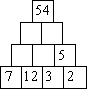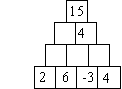What are the missing numbers?

(ii)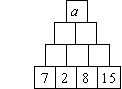What is the value of a?

(iii)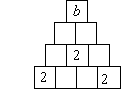Find the value of b for which the pyramid can be filled with whole numbers in this pattern. With this value of b find some different ways of doing so.

(iv)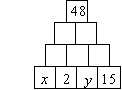Find eight different pairs of positive values of x and y . How many solutions are there altogether?

Can you find a relationship between x and y ?